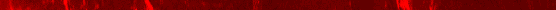# DML FAQDML FAQ : Transmission and Gears : How can I calculate my speed from engine RPM?

## Q: How can I calculate my speed from engine RPM?

This should get you close:

(RPM * tire height * .00297) / (rearend ratio * tranny ratio)
For example, a truck with 28" tall tires and 3.55s in 4th gear at 3,000 RPM:
(3000 * 28 * .00297) / (3.55 * 1) = 70.3mph
Tranny ratios can be found in the FSM, and some are in the DML FAQ also. Just use the number to the left of the colon. For example, an overdrive ratio of 0.80:1 is just .8, a ratio of 3.42:1 is just 3.42).
Contributed by:
Last updated: Sun Oct 13 09:23:52 2002